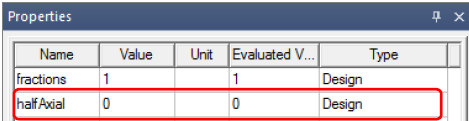## Electronics

Topics relate to HFSS, Maxwell, SIwave, Icepak, Electronics Enterprise and more

•M_reda
Subscriber
•Navya C
Ansys Employee

Hi @M_reda
The assumption when using 2D cross-section to represent a 3D model is that the fields are evenly distributed along the axial direction. This assumption is reasonable when the stack length of the machine is long enough such that the end effect of 3D model can be neglected. However, if your machine has a short stack length the assumption does not match the actual model well so you will get that amount of difference. You will see the difference drops as the stack length increases.
The difference between 3D and 2D model are mainly the end winding effect and the fringe effect in stator/rotor cores. If you have a short stack length it is likely that the difference can not be ignored. The difference is the result of the base assumption of 2D rather than the result of your settings.
So I would suggest just use 2D simulation for rough calculation and use 3D for fine-tuning
For your second Question: Select 3D model design, and in the properties window make half Axial value to zero, to get a full machine model in 3D.Regards
Navya
•M_reda
Subscriber